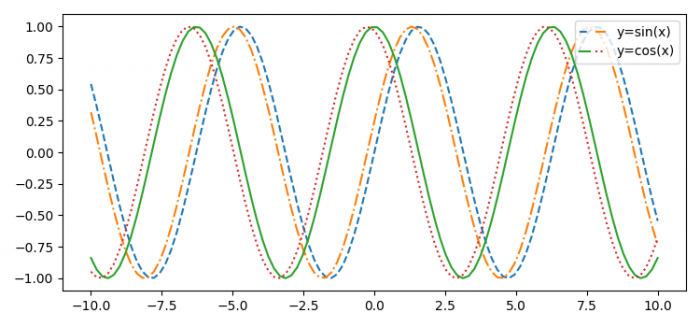# How to set a title above each marker which represents a same label in Matplotlib?

MatplotlibPythonData Visualization

To set a title above each marker which represents the same label in Matplotlib, we can take the following steps −

• Set the figure size and adjust the padding between and around the subplots.

• Create x data points using Numpy.

• Create four curves, c1, c2, c3 and c4 using plot() method.

• Place a legend on the figure, such that the same label marker would come together.

• To display the figure, use show() method.

## Example

import numpy as np
from matplotlib import pyplot as plt, legend_handler

plt.rcParams["figure.figsize"] = [7.50, 3.50]
plt.rcParams["figure.autolayout"] = True

x = np.linspace(-10, 10, 100)

c1, = plt.plot(x, np.sin(x), ls='dashed', label='y=sin(x)')
c2, = plt.plot(x, np.sin(x+0.25), ls='dashdot', label='y=sin(x)')
c3, = plt.plot(x, np.cos(x), ls='solid', label='y=cos(x)')
c4, = plt.plot(x, np.cos(x+0.25), ls=':', label='y=cos(x)')

plt.legend([(c1, c2), (c3, c4)], ['y=sin(x)', 'y=cos(x)'], loc='upper right', handler_map={tuple: legend_handler.HandlerTuple(ndivide=None)})

plt.show()

## Output